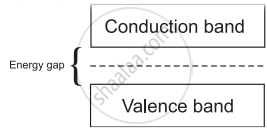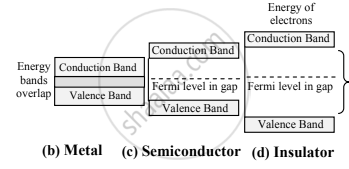# Answer in detail. Explain how solids are classified on the basis of the band theory of solids. - Physics

Explain how solids are classified on the basis of the band theory of solids.

#### Solution

1. The solids can be classified into conductors, insulators, and semiconductors depending on the distribution of electron energies in each atom.
2. As an outcome of the small distances between atoms, the resulting interaction amongst electrons, and Pauli’s exclusion principle, energy bands are formed in the solids.
3. In metals, the conduction band, and valence band overlap. However, in a semiconductor or an insulator, there is a gap between the bottom of the conduction band and the top of the valence band. This is called the energy gap or bandgap.(a) Energy bands for a typical solid
4. For metals, the valence band, and the conduction band overlap, and there is no band gap as shown in figure (b). Therefore, electrons can easily gain electrical energy when an external electric field is applied and are easily available for conduction.
5. In the case of semiconductors, the band gap is fairly small, of the order of 1 eV or less as shown in figure (c). Hence, with the application of an external electric field, electrons get excited and occupy energy levels in the conduction band. These can take part in conduction easily.
6. Insulators, on the contrary, have a wide gap between the valence band and conduction band of the order of 5 eV (for diamond) as shown in figure (d). Therefore, electrons find it very difficult to gain sufficient energy to occupy energy levels in the conduction band.
7. Thus, an energy band gap plays an important role in classifying solids into conductors, insulators, and semiconductors based on the band theory of solids.Band Structure
Concept: Band Theory of Solids
Is there an error in this question or solution?

#### APPEARS IN

Balbharati Physics 11th Standard Maharashtra State Board
Chapter 14 Semiconductors
Exercises | Q 3. (i) | Page 256